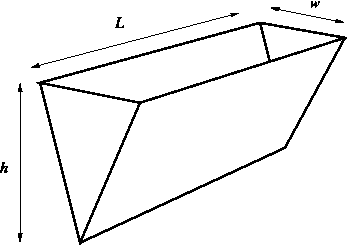The trough in the figure below has width $w = 2$ ft, length $L = 12$ ft and height $h = 4$ ft.(Click on the graph for a larger version.)

If the trough is full of water, find the force of the water on a triangular end. (Use the density of water $= 62.4\hbox{ lb/ft}^3$.)
Force =
(include units)

Find the work to pump all of the water over the top of the trough.
Work =
(include units)

You can earn partial credit on this problem.